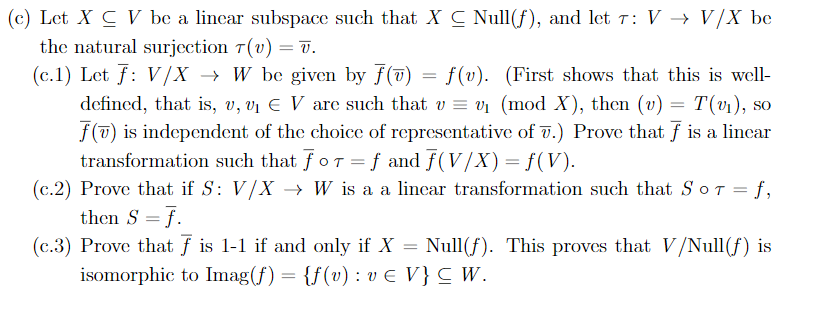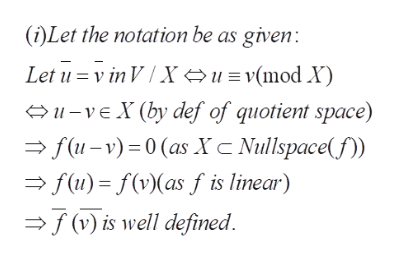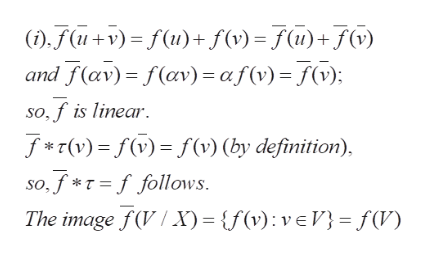# (c) Let X C V be a lincar subspace such that X C Null(f), and let T: V > V/X bethe natural surjcction T(v) = 7.(c. 1) Let f V/X W be given by f(7)f(v). (First shows that this is welldefincd, that is, v, vi E V are such that w = vi (mod X), then (u) = T(v1),f() is independent of the choice of representative of .) Prove that f is a lincartransformation such that foT =f and f(V/X) = f(V)(c.2) Prove that if S: V/X -> W is a a lincar transformation such that SoT = f,then S f(c.3) Prove that f is 1-1 if and only if Xisomorphic to Imag(f) {f(v): ve V}C W.SONull(f). This proves that V/Null (f) is

Question
60 views
Please, help me with very detailed and step by step solutions for my understanding of questions c1, c2, and c3. I will be much appreciative of your solutions. Thank you

This question is about Quotient space: Let V and W be vector spaces over a field K and f:V→W a linear map.help_outlineImage Transcriptionclose(c) Let X C V be a lincar subspace such that X C Null(f), and let T: V > V/X be the natural surjcction T(v) = 7. (c. 1) Let f V/X W be given by f(7)f(v). (First shows that this is well defincd, that is, v, vi E V are such that w = vi (mod X), then (u) = T(v1), f() is independent of the choice of representative of .) Prove that f is a lincar transformation such that foT =f and f(V/X) = f(V) (c.2) Prove that if S: V/X -> W is a a lincar transformation such that SoT = f, then S f (c.3) Prove that f is 1-1 if and only if X isomorphic to Imag(f) {f(v): ve V}C W. SO Null(f). This proves that V/Null (f) is fullscreen
check_circle

Step 1

(i) The idea is to factor the given linear map f from V to W, using the natural quotient map t( tau) . This is possible because X is contained in the null space of fhelp_outlineImage Transcriptionclose(i)Let the notation be as given Let u v in VXu= v(mod X) 1vEX (by def of quotient space) f(u-v) 0 (as XC Nullspace f(u)f(vas f is linear) (v) is well defined fullscreen
Step 2

Proof of (i) completedhelp_outlineImage Transcriptionclose),(+v)= f(u)+ f(v)=(u)+f(v) and f(av) f(av) = af (v) (v); so,f is linear T(v)f (v) (by definition), so,f*=ffollows The image f (V/X) = {f(v) veV= f(V) fullscreen
Step 3

Proof of (...

### Want to see the full answer?

See Solution

#### Want to see this answer and more?

Solutions are written by subject experts who are available 24/7. Questions are typically answered within 1 hour.*

See Solution
*Response times may vary by subject and question.
Tagged in

### Math